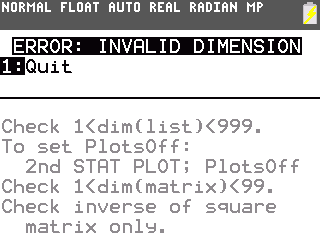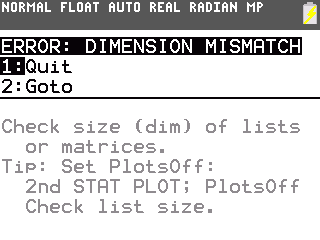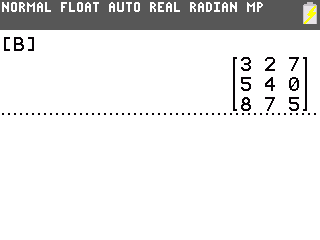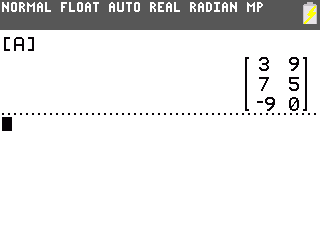# Knowledge Base

## Solution 34573: Resolving an ERR:INVALID DIM or ERR:DIM MISMATCH Error on the TI-83 Plus and TI-84 Plus Family of Graphing Calculators.

### How do I resolve an ERR:INVALID DIM and ERR:DIM MISMATCH error on the TI-83 Plus and TI-84 Plus family of graphing calculators?

An ERR:INVALID DIM error is returned if one or more STAT PLOTS is turned ON but there are no values in the STAT lists.

An ERR:DIM MISMATCH error is returned when performing an operation that references one or more lists or matrices whose dimensions do not match.

For example, trying to graph a scatter plot when there are no values stored in the L1 and L2 lists (or other lists) will result in the error displayed below:For example, trying to calculate a 2-Var Statistics when there are four values in L1 and five values in L2 will result in the error message displayed below:If using matrices, to multiply two matrices ([B]*[A]), the column dimension of Matrix [B] must be equal to the row dimension of Matrix [A]. If the dimensions do not match, an ERR:DIM MISMATCH error will be displayed.

Matrix [B]:Matrix [A]:Using the example matrices above, matrix B is a 3 X 3 and matrix A is a 3 X 2. Matrix B can be multiplied by matrix A ([B]*[A]); however, matrix A cannot be multiplied by matrix B because the column dimension (2) in matrix A is not equal to the row dimension (3) in matrix B.

If either error occurs when trying to graph a function (not a STAT PLOT), the error can be corrected by turning off the STAT PLOTS. The following steps will turn the STAT PLOTS off, allowing the graph of the function to be displayed.

1) Press [2nd] [STAT PLOT] to access the STAT PLOTS menu.
2) Select 4:PlotsOff.
3) Press [ENTER].
4) Try graphing a simple function like y = x + 1.

If the above steps do not resolve the issue, try resetting the memory of the calculator.

Please Note: The reset below is called an All RAM memory reset, which will erase all programs, games, notes, data and functions stored by the user.

1) Press [2nd] [MEM] to access the Memory menu.
2) Press 7:Reset.
3) Press 1:All RAM.
4) Press 2:Reset to confirm the reset. When completed, RAM cleared will be displayed.

Please see the TI-83 Plus and TI-84 Plus Family guidebooks for additional information.